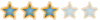# [分享]畫圖任意角度輔助線(建構線)，並繪於TEMP圖層，該圖層設為不出圖##[分享]畫圖任意角度輔助線(建構線)，並繪於TEMP圖層，該圖層設為不出圖

`;XAN.lsp;作圖線-任意角度(defun C:XAN()  (graphscr)  (setvar "CMDECHO" 0);===作圖線建構於TEMP圖層===  (command "-LAYER" "P" "N" "0" "")  (setq LAY_CURRENT (getvar "CLAYER"))  (command "-LAYER" "ON" LAY_CURRENT "T" LAY_CURRENT "U" LAY_CURRENT "")  (setq LL (tblsearch "LAYER" "TEMP"));偵測是否有TEMP圖層  (if (= LL nil)(command "-LAYER" "N" "TEMP" ""));若無則新建TEMP圖層  (command "-LAYER" "ON" "TEMP" "T" "TEMP" "U" "TEMP" "C" "47" "TEMP"  "P" "N" "TEMP""")  ;打開TEMP並解鎖,解凍,設為不出圖;===  (setq    OSP (getvar "OSMODE")    OCT (getvar "CTAB")    OCV (getvar "CVPORT")  )  (setq PT1 (getpoint "\n作圖線第一點："))  (while (/= PT1 nil)    (command "UNDO" "BE")    (princ "\n作圖線另一點 [Esc 離開]：")    (command ".XLINE" PT1 pause "")    (setq PT2 (getvar "LASTPOINT"))    (setq P1 (polar PT1 (/ (* 135 PI) 180) 10))    (setq P2 (polar PT1 (/ (* 315 PI) 180) 10))    (setvar "OSMODE" 0)    (if (or (= OCT "Model")(= OCV 1))(command "ZOOM" P1 P2))    (setq PTN (ssget PT1))    (if (/= PTN nil)      (command "CHANGE" PT1 "" "P" "LA" "TEMP" "C" "BYLAYER" "LT" "BYLAYER" "")    )    (if (or (= OCT "Model")(= OCV 1))(command "ZOOM" "P"))    (setvar "OSMODE" OSP)    (command "UNDO" "E")    (setvar "OSMODE" 4151)    (setq PT1 (getpoint "\n作圖線第一點："))  )  (prin1))`djh1006##回復： [分享]畫圖任意角度輔助線(建構線)，並繪於TEMP圖層，該圖層設為不出圖##回復： [分享]畫圖任意角度輔助線(建構線)，並繪於TEMP圖層，該圖層設為不出圖

~謝謝分享~受益了~meet_emily